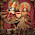# Java program to calculate Simple Interest - homework exercise

How to calculate simple interest in Java or Write a Java program to calculate Simple interest is a popular Java programming exercise people use in Java course in school and college. So if you have recently got an homework exercise about calculating Simple interest in Java and wondering How to write Java program than this tutorial can help you, provided you know What is simple interest and What is formula of simple interest. If you are completely new in Java programming than I would suggest to try HelloWorld Example in Java and understand What is Path and Classpath in Java. IF you have been doing Java programming and looking for some good coding exercise than you can also see our earlier programs e.g. calculating Fibonacci series using recursion, checking if number is palindrome and How to reverse String in Java using recursion.

## How to calculate Simple Interest in Java

Here is full code example of Java program to calculate Simple Interest in Java. This is an interactive Java program which accept Input from User using java.util.Scanner class. By the way simpleInterest() method is reusable method and I have made it static so that I can call it from main method in Java without creating object of this class. Its good Java practice to make utility method static./**
*
* Java program to calculate Simple Interest in Java.
* Input to program is principle, rate and time and output is simple interest
* @author
*/

public class SimpleInterestTest{

public static void main(String args[]) {

//creating scanner to accept principle, rate and time input form user
Scanner scanner = new Scanner(System.in);
System.out.println("Welcome in Java program to calculate Simple interest");

float amount = scanner.nextFloat();

System.err.println("Enter time in years : ");
float time = scanner.nextFloat();

System.out.println("Enter rate annually : ");
float rate = scanner.nextFloat();

float interest = simpleInterest(amount, rate, time);

System.out.println("Simple interested calculate by program is : " + interest);
}

public static float simpleInterest(float principle, float rate, float time){
float interest = (principle*rate*time)/100;
return interest;
}
}

Output:
Welcome in Java program to calculate Simple interest
1000
Enter time in years :
1
Enter rate annually :
7
Simple interested calculate by program is : 70.0

So we saw we have create a static method to calculate simple interest for given amount, rate and time. By the way this Java program is not validating input e.g. time must be greater than zero, rate should be more than zero, amount can not be zero or negative, which will be require if you are writing production code.

Further Learning
Data Structures and Algorithms: Deep Dive Using Java
Java Fundamentals: The Java Language
Complete Java Masterclass

Some more Java Coding Exercise for Programmer

1.Nice one. Thanks for sharing your knowledge regarding the formula for calculating simple interest .

2.i have one doubt, this output amount is per month rate or per year rate.

3.per year rate not per month...

4.5.That's interesting post.

6.Can you please post a Java program to calculate compound interest as well, please make interest is compounded quarterly or half yearly or yearly.

thanks

1.@Anonymous, sure we'll post program to calculate compound interest in Java shortly.

2.import java.util.Scanner;

public class CurrentWorthCalculator
{
public static void main(String[] args)
{
double a=0;
double r=0;
double n=0;
double x=0;

Scanner input ;

System.out.println("Welcome in Java program to calculate Present Value");
input = new Scanner(System.in);

a= input.nextDouble();

System.out.print("Please enter the rate of inflation/interest(in %):");
r= input.nextDouble();

System.out.println("Please enter the time period (in years)");
n= input.nextDouble();

x= a / Math.pow((1 + r/100),n);

System.out.println("Present Value is : " + x);

}
}

To calculate reverse compound int/ present value

3.hello @Anonymous, does the present value is equal to compound interest, don't you need to subtract principle to print compound interest?

7.nice ones but can u make a program for simple interest with a fixed numericals

8.9.I want know that can we do this programme using object oriented programming method?

10.can we do this program by comparing simple Interest of two different banks using packages?